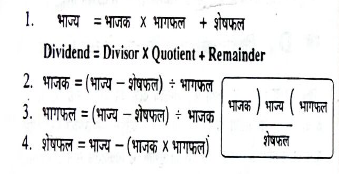Hello friends today we are sharing an e book of formula for Competitive exam .In this book you can get all useful formulas from Arithmetic,Algebra,Geometry,Mensuration,Trigonometry .We hope this may be helpful for you . As maths is a common part of every competitive examination like SSC,IBPS,Railways.This book has formula from basic level to advanced level. The contents list of this book is given  below .Please find download link below.Formula eBook

Description:
Book Type - eBook
File Size-8 MB
File Type-PDF

Contents :
• Signs used in mathematics

Arithmetic

• Type of numbers
• Metric system
• number system
• Average
• Division
• LCM and HCF
• Time and Distance
• Ratio and Proportional
• BODMAS
• Simple Interest
• Compound Interest
• Loss and Profit

Algebra

• Law of Subtraction
• Law of Multiplication
• Law of division
• Law of Indices
• Law to solve In equation

Geometry

• Definitions of all geometry

Mensuration

• Rectangle
• Square
• Right angle Triangle
• Equilateral Triangle
• Isosceles Triangle
• Scalene Triangle
• Parallelogram
• Trapezium
• Rhombus
• Circle
• Rectangular Parallelepiped
• Cube
• Right circular Cylinder
• Pyramid
• Right Circular Cone
• Sphere

Trigonometry

• General formula